##### Differential Equations For Dummies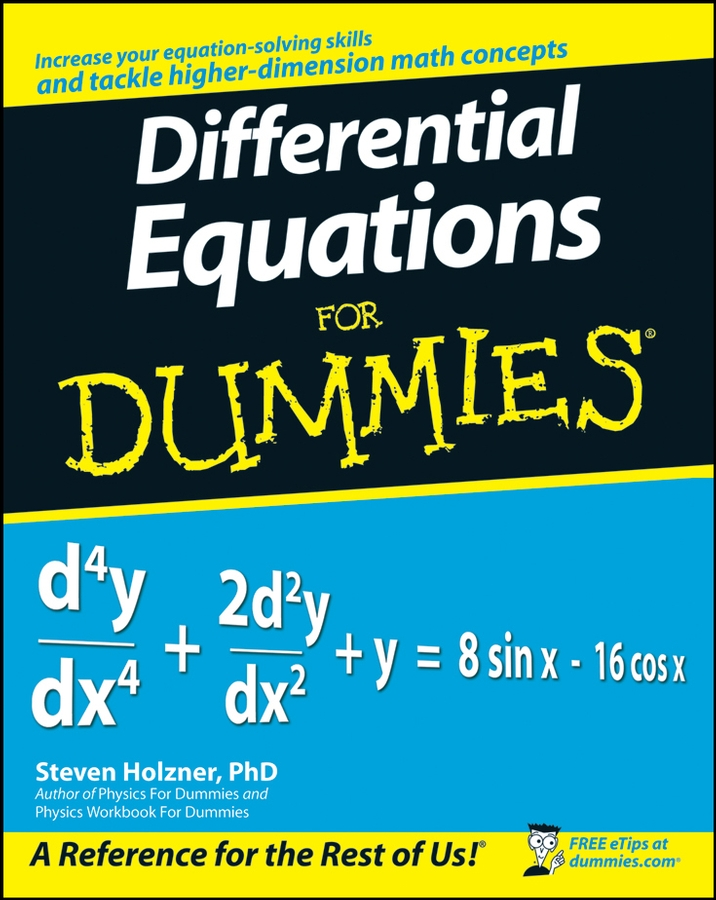When you tackle calculus problems involving position, velocity, and acceleration, it's important to know how these three vectors relate to each other. Remember that velocity is the derivative of position, and acceleration is the derivative of velocity.

The following practice questions ask you to find the position, velocity, speed, and acceleration of a platypus in relation to a boat he is swimming around.

## Practice questions

For the following two practice questions, a duck-billed platypus is swimming back and forth along the side of your boat, blithely unaware that he's the subject for calculus problems in rectilinear motion. The back of your boat is at the zero position, and the front of your boat is in the positive direction (as shown in the figure). s(t) gives the platypus's position (in feet) as a function of time (seconds).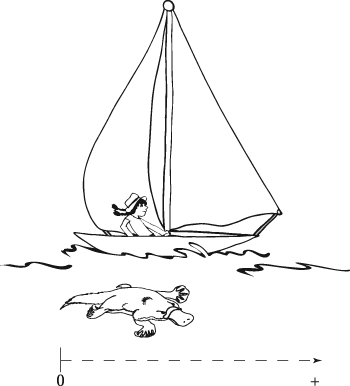Referring to the figure, find the a) position, b) velocity, c) speed, and d) acceleration of the platypus at t = 2 seconds based on the following position equations.

1. Find s(t) = 3t4 – 5t3 + t – 6

2. 2. Find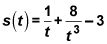## Answers and explanations

1. 1. Here is the answer broken down:

a. position: s(2) gives the platypus's position at t = 2 ; that's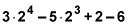or 4 feet, from the back of the boat.

b. velocity: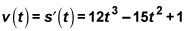At t = 2, the velocity is thus 37 feet per second.

c. speed: Speed is also 37 feet per second.

d. acceleration: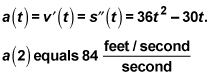2. Here is the answer broken down:

a. position: At t = 2, s(2) equals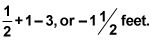This means that the platypus is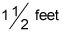behind the back of the boat.

b. velocity: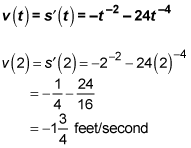A negative velocity means that the platypus is swimming "backwards," in other words, he's swimming toward the left, moving away from the back of the boat.

c. speed: Speed = |velocity|, so the platypus's speed is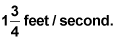d. acceleration: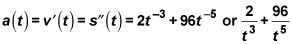a(2) is therefore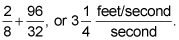Give yourself a pat on the back if you figured out that this positive acceleration with a negative velocity means the platypus is actually slowing down.